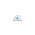### Electrical Terms

In this article, I will share 3 formula and give some definitions. (volt =V, ampere=I, resistance=R, power=P, electric energy=E , time=t)

V = I * R         &           P (watt) = I * V = V^2 / R         &          E (joule) = V * I * t  = P * t

(dbm = decibek-miliwatts; it is a unit of power. It increases logarithmic.)

• Everything including yourself has resistance (also called impedance) to electricity. Its measurement is ohm.
• Everything occurs from electrons. Elektrik akımını taşıyacak serbest elektronlara sahip olmayan maddelere yalıtkan maddeler denir.

1.